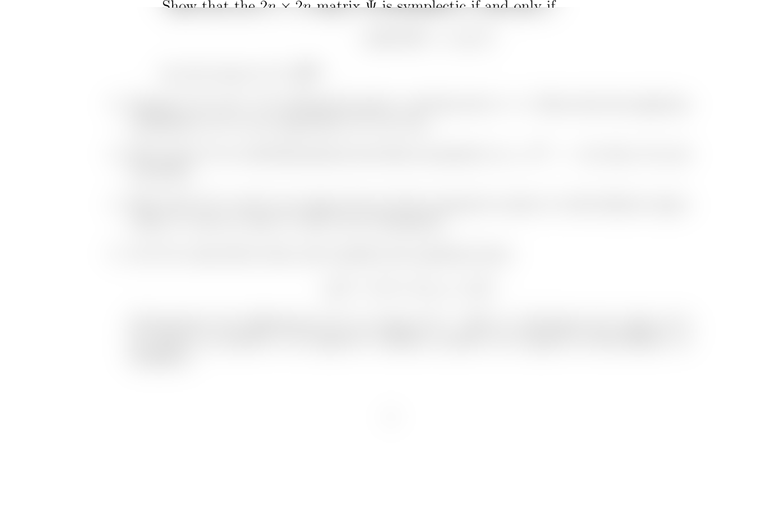Study Guides (380,000)
US (220,000)
UCLA (3,000)
MATH (300)
All (20)
Final

MATH 33AH Lecture Notes - Lecture 22: Symplectic Matrix, Symmetric Matrix, Block MatrixExam

Department
Mathematics
Course Code
MATH 33AH
Professor
All
Study Guide
Final

This preview shows half of the first page. to view the full 1 pages of the document.Math 33A Practice Problems
November 30, 2016
Here you’ll ﬁnd a few practice problems for the exam. It’s important to understand
that these problems were not vetted by Dr. Shlyakhtenko, and should not be
considered an indication of the problems you’ll see on the exam.
1. Let J0be the following 2n×2nblock matrix:
J0=0In
In0.
A 2n×2nmatrix Ψ is called symplectic if ΨTJ0Ψ = J0.
(a) Show that all symplectic matrices have determinant ±1.
(b) Show that if Ψ and Φ are symplectic matrices, then so are Ψ + Φ, ΨΦ, Ψ1, and
ΨT.
(c) Characterize all 2 ×2 symplectic matrices.
(d) Consider the map ω:R2n×R2nRdeﬁned by
ω(~u, ~v) = ~uTJ0~v.
Show that the 2n×2nmatrix Ψ is symplectic if and only if
ω(Ψ~u, Ψ~v) = ω(~u, ~v)
for all vectors ~u, ~v R2n.
2. Suppose Ais a 2n×2northogonal matrix, and that det A= 1. Show that the algebraic
multiplicity of 1 as an eigenvalue of Ais even.
3. Show that if Ais odd-dimensional and skew-symmetric (i.e., AT=A), then Ais not
invertible.
4. Show that if ~v1and ~v2are eigenvectors of the symmetric matrix Awith distinct eigen-
values λ1and λ2, then ~v1and ~v2are orthogonal.
5. Let bbe some xed value and consider the quadratic form
q(~x) = kx2
1+bx1x2+kx2
2.
Characterize the deniteness of qin terms of k. That is, determine the values of k
for which qis positive- (or negative-) denite, positive (or negative) semi-denite, or
indeﬁnite.
1